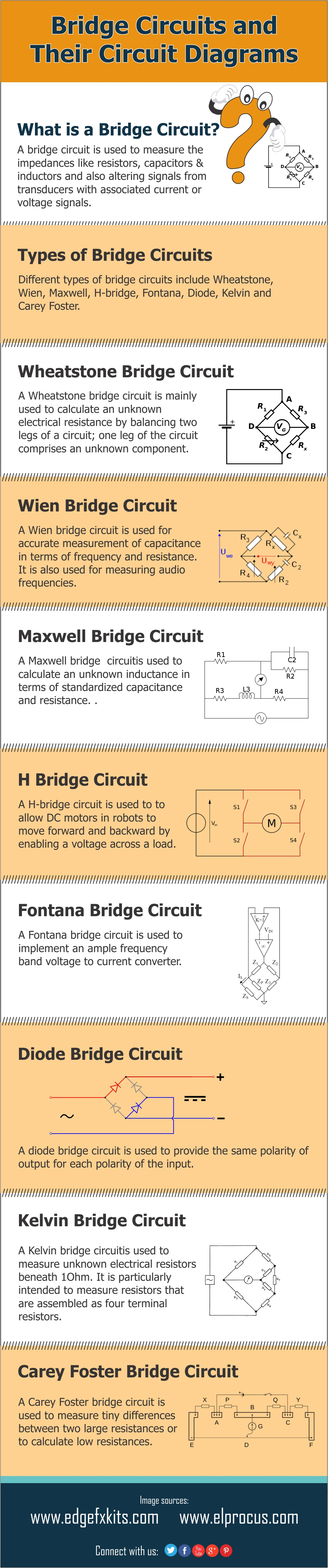# Infographics : Different Types of Bridge Circuits and Circuit Diagrams

A bridge circuit is one kind of electrical circuit wherein the two branches of the circuit are linked to a third branch –which is connected in between the first two branches at some middle point along with them. The bridge circuit was mainly designed for measurement purposes in the laboratory. And, one of the middle linking points is adjusted when it is used for a specific purpose. These circuits are used in linear, nonlinear, power conversion, instrumentation, filtering, etc.

The best-known bridge circuit is the Wheatstone bridge; the term was invented by “Samuel Hunter Christie” and popularized by “Charles Wheatstone”. A bridge circuit is mainly used to measure resistance. This circuit is built with four resistors: R1, R2, R3 and RX; wherein the two resistors are with known values R1 & R3, one resistor’s resistance is to be concluded Rx, and one which is changeable and adjusted R2. Two opposite vertices are associated with a supply of electric current like a battery, and a galvanometer is connected across the additional two vertices. The variable resistor is familiarized until the galvanometer reads zero.

It is known that the relation between the variable resistor and its neighbor resistor R1 is equivalent to the relation between the unknown resistor and its neighbor R3, which permits the unknown value of the resistor to be calculated. The Wheatstone bridge circuit has also been widespread to calculate impedance in AC circuits, and also to calculate inductance, resistance, capacitance, and dissipation factor individually.

Different arrangements are identified as Wien bridge, Heaviside, and Maxwell bridge. All the circuits are based on a similar concept, which is to contrast the o/p of two potentiometers sharing a frequent source.

## Bridge Circuits and their Circuit Diagrams

What is a Bridge Circuit?

A bridge circuit is used to measure the impedances like resistors, capacitors & inductors and also altering signals from transducers with associated current or voltage signals.

Types of Bridge Circuits

Different types of bridge circuits include Wheatstone, Wien, Maxwell, H-bridge, Fontana, Diode, Kelvin and Carey Foster.

Wheatstone Bridge Circuit

A Wheatstone bridge circuit is mainlyused to calculate an unknown electrical resistance by balancing two legs of a circuit; one leg of the circuit comprises an unknown component.

Wien Bridge Circuit

A Wien bridge circuit is used for accurate measurement of capacitance in terms of frequency and resistance. It is also used for measuring audio frequencies.

Maxwell Bridge Circuit

A Maxwell bridge circuit is used to calculate an unknown inductance in terms of standardized capacitance and resistance. .

H Bridge Circuit

A H-bridge circuit is used to allow DC motors in robots to move forward and backward by enabling a voltage across a load.

Fontana Bridge Circuit

A Fontana bridge circuit is used to implement an ample frequency band voltage to current converter.

Diode Bridge Circuit

A diode bridge circuit is used to provide the same polarity of output for each polarity of the input.

Kelvin Bridge Circuit

A Kelvin bridge circuit is used to measure unknown electrical resistors, beneath1 Ohm. It is particularly intended to measure resistors that are assembled as four-terminal resistors.

Carey Foster Bridge Circuit

A Carey Foster bridge circuit is used to measure tiny differences between two large resistances to calculate low resistances.Embed This Image On Your Site (copy code below):

<div style=”clear:both”><a href=”https://www.elprocus.com/different-types-bridge-circuits-and-circuit-diagrams/”><img src=”https://www.elprocus.com/wp-content/uploads/2016/08/Different-Types-of-Bridge-Circuits-and-Their-Circuit-Diagrams.jpg” title=”Different Types of Bridge Circuits and Circuit Diagrams” alt=”Different Types of Bridge Circuits and Circuit Diagrams” border=”0″ /></a></div><div>Courtesy of: <a href=”https://www.elprocus.com/different-types-bridge-circuits-and-circuit-diagrams/”>Elprocus.com</a></div>